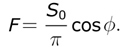# Exams

## Midterm Exam

1. Determine the emission temperature of the planet Venus. You may assume the following: the mean radius of Venus’ orbit is 0.72 times that of the Earth’s orbit; the solar flux _S_0 decreases as the square of the distance from the Sun and has a value of 1367W/m2 at the mean Earth orbit; Venus planetary albedo is 0.77. The observed mean surface temperature of the planet Venus is about 750K. How many layers of the N-layer model described in Lecture 4 (and in Problem 2.1 in the textbook) would be required to achieve the same degree of warming? Comment.

2. Let us consider a typical lecture of 12.003. There are 10 students, two TAs and one professor in a well-insulated classroom measuring 20 meters by 20 meters by 3 meters. Each person in the classroom puts out energy at a rate of 100 Watts (1 Watt = 1 Joule/second). The classroom is dark, except for a computer and LCD projector which together consume power at a rate of 200 Watts. The classroom is filled with air at a pressure of 1000hPa. How much does the temperature of the classroom rise during the course of a 1 hour lecture? Assume that at the beginning of the class the temperature was 290K. Neglect the specic heat of the walls, the furnishings, and the people in the room!

Hint: The specific heat of air, at constant volume, is 720 Joules/Kg.

3. The temperature profile of the tropical troposphere is maintained by moist convection in the form of heavily raining cumulonimbus cloud systems. The strongest updrafts lift moist air from near the surface to an altitude higher than 15km. They stay buoyant due to latent heating due to condensation in the rising, cooling updrafts. By the time they reach 15km, essentially all of their water vapor has condensed into liquid water or ice, which precipitates out of the updraft. This air spreads out to define the temperature just below the tropopause.

(a) Suppose the air starts at a near-surface pressure of _p_s = 1000hPa, temperature of 302K, and specic humidity q = 0.019 kg kg-1. Calculate the air density at this pressure. Here and throughout this problem, ignore the virtual effect of water vapor on the air density. Using a reference pressure _p_ref = 1000hPa, what is the potential temperatureof the air?

(b) Suppose this air is lifted to the top of the cumulonimbus clouds at an ambient pressure of _p_t = 125hPa, without any external input of heat. If the water all stayed as vapor, what would its potential temperature be? How about its temperature?

(c) If (more realistically) the water vapor condenses into cloud in the rising air parcel, this produces condensational heating of the rising air parcel. Suppose that as the air rises up to 125hPa, all of the water vapor is condensed into liquid water and rained out. This provides a heat input L per unit mass of air, where L = 2.5 x 106J kg-1 is the latent heat of condensation. This heat input takes place gradually as the air cools, but for simplicity assume that the heat is all added at a representative pressure _p_q = 700hPa. Argue that this will increaseof the rising air parcel by _Lq=(c_pq ), where ∏q = _(p_q _⁄ p_ref _)_K. What will its potential temperaturet now be at 125hPa? What will its temperature and density be?

4. Suppose that the Earth’s rotation axis were normal to the Earth-Sun line. The solar flux, measured per unit area in a plane normal to the Earth-Sun line, is _S_0. By considering the solar flux incident on the latitude belt bounded by latitudes (Ø, Ø + dØ), show that F, the 24-hr averaged flux per unit area of the Earth’s surface, varies with latitude as,(1)

Using this result, suppose that the atmosphere is completely transparent to solar radiation such that, separately at each latitude, the radiation budget can be represented by the single slab model shown in Fig. 2.7 of the textbook. Determine how surface temperature varies with latitude.

5. [Bonus point] What topic did you find most interesting in the first part of the class? And which one was the least interesting?

The midterm exam is to be done by you as an individual: no group efforts. You can either print or handwrite your answers, but make sure that the text is neatly written. Full credit requires proper units be included. Any plot must be completely and unambiguously labeled, including title and axes. Show ALL math steps. Good luck! (PDF)

## Final Exam

Final exam is not posted due to copyright restrictions.

#### Learning Resource Types

theaters Demonstration Videos
laptop_windows Simulations
assignment Problem Sets